# Overview¶

HARK-Binaural+ includes binaural signal processing nodes for robot audition software HARK. You can also smoothly connect HARK-Binaural+ and other HARK packages such as HARK-FD or HARK-SSS packages.

# Getting Started¶

1. Add HARK repository and install Basic HARK Packages. See HARK installation instructions for details.

2. Install HARK-Binaural+

sudo apt-get install hark-binaural+


# Binaural Signal Processing Nodes¶

This section describes following 5 signal processing nodes for binaural robot audition:

1. BinauralMultisourceLocalization node
2. BinauralMultisourceTracker node
3. SourceSeparation node
4. SpeechEnhanvement node
5. VoiceActivityDetection node

## BinauralMultisourceLocalization Node¶

### Outline of the node¶

This node identify the location of sound sources in direction with two microphones.

### Typical connection¶

The type of the input is multi-channel (2-ch) audio spectrum and that of the output is a list of localized directions of sound sources. Typical connection of this node is depicted as follows: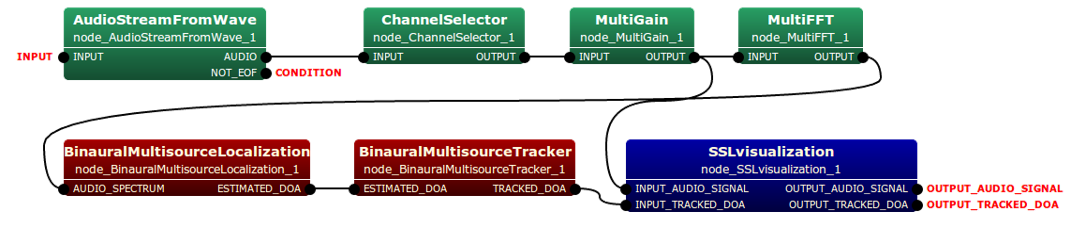### Input-output and property of the node¶

#### Input¶

AUDIO_SPECTRUM Matrix<complex<float> >
Windowed spectrum data. A row index is channel, and a column index is frequency.

#### Output¶

ESTIMATED_DOA Matrix<complex<float> >
Estimated directions of multi-sources.

#### Parameters¶

Parameters of this node are listed as follows:

Parameter name Type Default value Unit Description
SOUND_DIFFRACTION_COMPENSATION string FREE_SPACE   Compensation for the diffraction of sound waves with multi-path interference caused by contours of a spherical robot head.
GCCPHAT_THRESHOLD float 0.25   Threshold to avoid estimating DOAs of noise and reverberated sources.
MICS_DISTANCE float 17.4 cm Distance between two microphone.
NOISE_DURATION float 1.0 second Time duration to be regarded as “noise” from the first frame.
ADVANCE int 160 sample The length in sample between a frame and a previous frame.
SAMPLING_RATE int 16000 Hz Sampling rate.

### Detail of the node¶

This node localizes sound sources based on generalized cross-correlation method weighted by the phase transform (GCC-PHAT) . Although the basic GCC-PHAT algorithm assumes the number of sound sources is one, we maintain multiple sound sources by using dynamic K-means clustering  that is implemented in BinauralMultisourceTracker node. The localization performance is improved by signal-to-noise ratio (SNR)-weighting .

Let $$\hat{\Theta}_{mel} = \{\hat{\theta}_1, \hat{\theta}_2, \hat{\theta}_3, \cdots\}$$ be directions of sound sources localized, the binaural sound source localization is conducted following process:

$$\hat{\Theta}_{mel} = \mathop{\rm argmax}_{\theta} \frac{1}{F} \sum^F_{f=1} \frac{SNR_{inst}[f,n]}{1 + SNR_{inst}[f,n]} \cdot \frac{X_l[f,n]X_r^{\ast}[f,n]}{\left|X_l[f,n]X_r^{\ast}[f,n]\right|} \exp{\left( j2 \pi \frac{f}{F} fs \tau_{multi}(\theta) \right)},$$

$$SNR_{inst}\left[f,n\right]=\frac{\left|X_l\left[f,n\right]X_r^{\ast}\left[f,n\right]\right|-E\left[\left|N_l\left[f,n\right]N_r^{\ast}\left[f,n\right]\right|\right]} {E\left[\left|N_l\left[f,n\right]N_r^{\ast}\left[f,n\right]\right|\right]}$$, and

$$\tau_{multi}(\theta)=\frac{d_{lr}}{2v} \left( \frac{\theta}{180}\pi + {\rm sin}\left( \frac{\theta}{180}\pi \right) \right) - \frac{d_{lr}}{2v}\left({\rm sgn}(\theta)\pi - \frac{2\theta}{180}\pi \right) \cdot \left|\beta_{multi} {\rm sin}\left( \frac{\theta}{180}\pi\right)\right|$$

where $$X\left[f, n\right]$$ represents an input audio signal at frequency bin $$f$$ and time frame $$n$$.

# Visualization Nodes¶

This section describes following 4 visualization nodes for the previous 5 nodes.

1. SSLVisualization node
2. SpectrumVisualization node
4. WaveVisualization node

## SSLvisualization Node¶

### Outline of the node¶

This node visualizes the estimated sound source locations.

### Typical connection¶

See Typical connection of the BinauralMultisourceLocalization node. This node can be connected with BinauralMultisourceTracker node. The following figure shows an example of the visualization.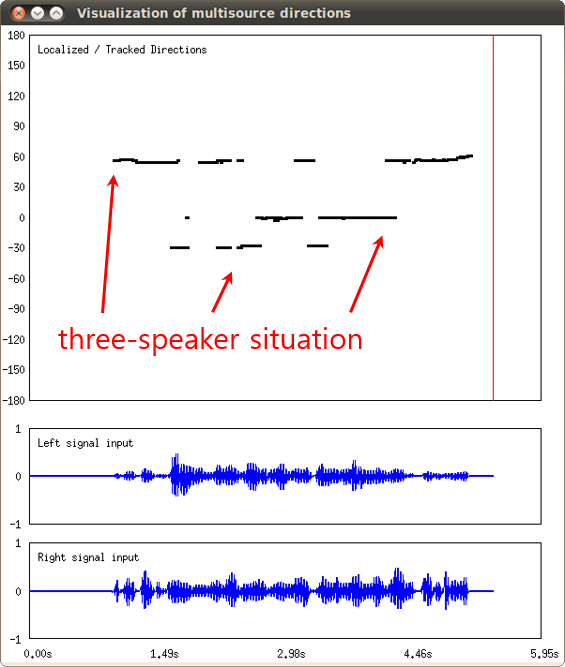### Input-output and property of the node¶

#### Input¶

INPUT_AUDIO_SIGNAL any
Input should be Matrix<float> or Map<int,ObjectRef>. In case of Matrix<float>, the rows should be channel indices and the columns should be frequency indices. In case of Map<int,ObjectRef>, the key is a source ID and the value is a vector of audio signals (Vector<float>).
INPUT_TRACKED_DOA Vector<ObjectRef>
Estimated/tracked directions of multisource.

#### Output¶

OUTPUT_AUDIO_SIGNAL any
Same as INPUT_AUDIO_SIGNAL
OUTPUT_TRACKED_DOA Vector<ObjectRef>
Same as INPUT_TRACKED_DOA

#### Parameters¶

Parameters of this node are listed as follows:

Parameter name Type Default value Unit Description
WINDOW_NAME string Visualization of multisource directions   Window name of the time-azimuth map.
VISUALIZATION_TIME_LENGTH float 10.0 second Visualization window length to show at the same time.
ADVANCE int 160 sample The length in sample between a frame and a previous frame.
SAMPLING_RATE int 16000 Hz Sampling rate.

## SpectrumVisualization Node¶

### Outline of the node¶

This node visualizes the audio spectrum.

### Typical connection¶

See Typical connection of the Source Separation node. Following figure shows the visualization result of spectrum on the input signal.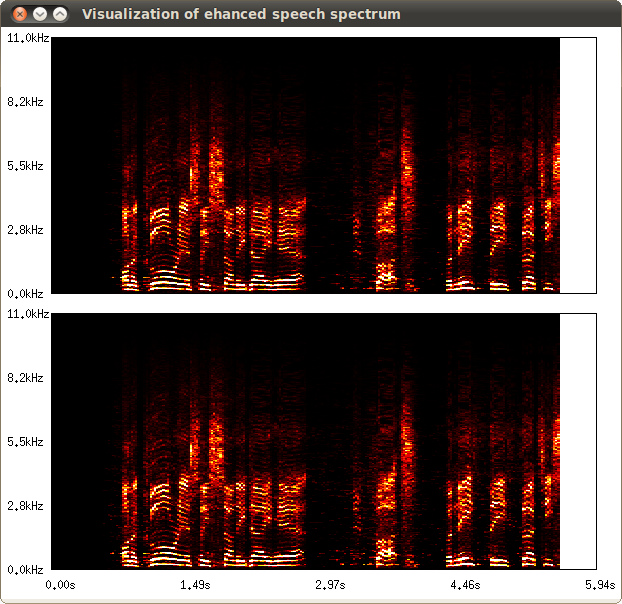### Input-output and property of the node¶

#### Input¶

AUDIO_SPECTRUM Matrixd<complex<float> >
Windowed spectrum data. A row index is channel, and a column index is frequency.

#### Output¶

AUDIO_SPECTRUM Matrix<complex<float> >
Same as INPUT_AUDIO_SIGNAL

#### Parameters¶

Parameters of this node are listed as follows:

Parameter name Type Default value Unit Description
WINDOW_NAME string Visualization of audio spectrum   Visualization of audio spectrum.
VISUALIZATION_TIME_LENGTH float 10.0 second Visualization window length to show at the same time.
ADVANCE int 160 sample The length in sample between a frame and a previous frame.
SAMPLING_RATE int 16000 Hz Sampling rate.

### Outline of the node¶

This node visualizes the detected speech segments.

### Typical connection¶

See Typical connection of the VoiceActivityDetection node. Following figure shows the visualization result of VAD overlayed on the input signal.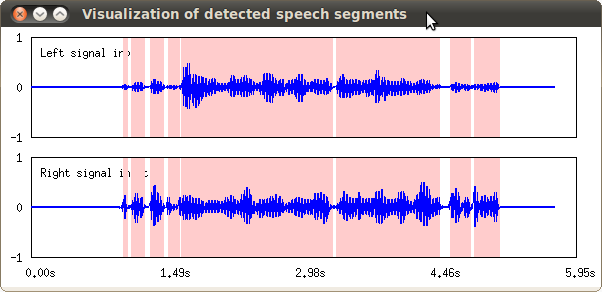### Input-output and property of the node¶

#### Input¶

INPUT_AUDIO_SIGNAL Matrix<complex<float> >
Input should be Matrix<float> of Map<int,ObjectRef>. In case of Matrix<float>, the rows should be channel indices and the columns should be frequency indices. In case of Map<int,ObjectRef>, the key is a source ID and the value is a vector of audio signals (Vector<float>).
Decision of speech-present frame.

#### Output¶

OUTPUT_AUDIO_SIGNAL any
Same as OUTPUT_AUDIO_SIGNAL.

#### Parameters¶

Parameters of this node are listed as follows:

Parameter name Type Default value Unit Description
WINDOW_NAME string Visualization of detected speech segments   Window name of the time-azimuth map.
VISUALIZATION_TIME_LENGTH float 10.0 second Visualization window length to show at the same time.
ADVANCE int 160 sample The length in sample between a frame and a previous frame.
SAMPLING_RATE int 16000 Hz Sampling rate.

## WaveVisualization Node¶

### Outline of the node¶

This node visualize the signal wave.

### Typical connection¶

The type of both the input and output of SourceSeparation node is multi-channel (2-ch) audio spectrum. Typical connection of this node is depicted as follows: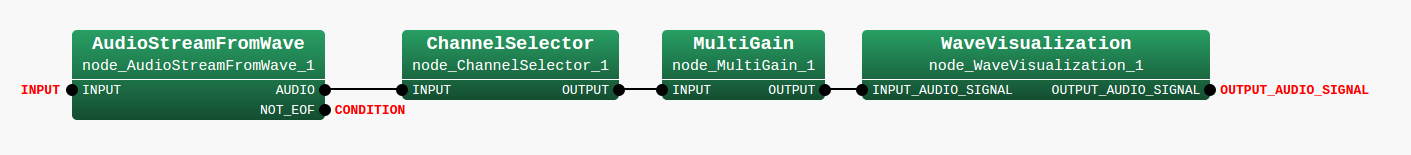The following figure shows the example of the result.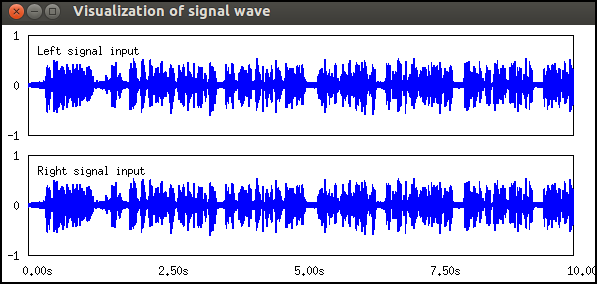### Input-output and property of the node¶

#### Input¶

INPUT_AUDIO_SIGNAL any
Input should be Matrix<float> or Map<int,ObjectRef>. In case of Matrix<float>, the rows should be channel indices and the columns should be frequency indices. In case of Map<int,ObjectRef>, the key is a source ID and the value is a vector of audio signals (Vector<float>).

#### Output¶

OUTPUT_AUDIO_SIGNAL any
Same as input.

Parameter list of

Parameter name Type Default value Unit Description
WINDOW_NAME string Visualization of detected speech segments   Window name of the time-azimuth map
VISUALIZATION_TIME_LENGTH float 10.0   Visualization window length to show at the same time
ADVANCE int 160   The length in sample between a frame and a previous frame
SAMPLING_RATE int 16000 Hz Sampling rate